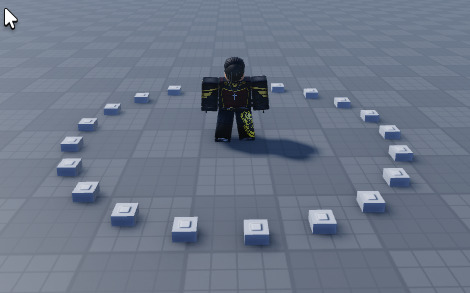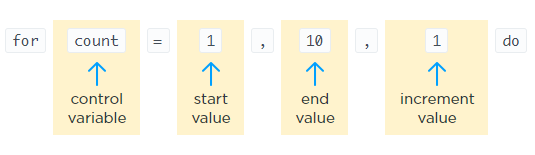# How to shoot rays in a spherical shape

I want to create a ray tracer and I need to shoot from a part rays in a spherical shape aka i need to shoot rays in spherical like directions but I cant because well I suck at math. I tried creating a loop for x, y and z axis from -1 to 1 with .1 increment and create a ray with that direction and multiply the direction vector with a distance but that didn’t work at all. Can someone tell me how I can create such a spherical shape of rays?

1 Like

if you want to shoot Ray’s in circle you simple do this in a loop:

``````x = Origin + cos(i) * RADIUS
z = Origin + sin(i) * RADIUS
``````6 Likes

said in a spherical (3d) not in a circle (2d) but can i make it like

``````x = Origin + cos(i) * RADIUS
z = Origin + sin(i) * RADIUS
y = Origin + something(i) * RADIUS
``````

can i make it like that? and if yes what this ‘something’ should be
btw what this i should be in the brackets?

Yes, I’ve understood perfectly what you mean, unfortunately, I’m not advanced enough with math so a circle is all I can script related to a sphere.

The “i” is the control variable of a for loop, you’ll need it to increase the circle, for the end value I would recommend using tau for a full circle which is 2pi (math.pi * 2) and the increment value used in the image is 0.3.1 Like